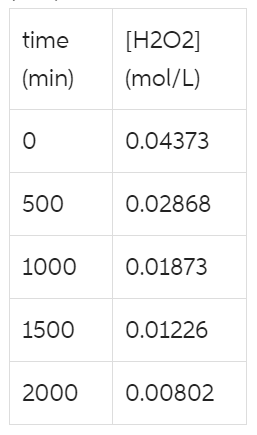# Problem: You will need to generate a set of graphs to answer this question. Concentration vs time data for the following decomposition reaction was collected at 300 K. The data is shown in the table below. What is the rate constant for the reaction at this temperature (in units of min-1)? H2O2(aq) → H2O(l) + 1/2 O2(g) time (min)

###### FREE Expert Solution

We're being asked to determine the rate constant for the reaction at this temperature (in units of min-1).

Step 1: Determine the order of the reaction. We can find the order of the reaction using the unit of the rate constant:

$\overline{){\mathbf{k}}{\mathbf{=}}{{\mathbf{M}}}^{\mathbf{-}\mathbf{n}\mathbf{+}\mathbf{1}}{\mathbf{·}}{{\mathbf{t}}}^{\mathbf{-}\mathbf{1}}}$

n = overall order of the reaction
n = order with respect to A

Given: unit of k = min−1.

t = minute, min

Recall that anything raised to zero is equal to 1:

99% (334 ratings)###### Problem Details

You will need to generate a set of graphs to answer this question. Concentration vs time data for the following decomposition reaction was collected at 300 K. The data is shown in the table below. What is the rate constant for the reaction at this temperature (in units of min-1)? H2O2(aq) → H2O(l) + 1/2 O2(g) time (min)Frequently Asked Questions

What scientific concept do you need to know in order to solve this problem?

Our tutors have indicated that to solve this problem you will need to apply the Integrated Rate Law concept. You can view video lessons to learn Integrated Rate Law. Or if you need more Integrated Rate Law practice, you can also practice Integrated Rate Law practice problems.